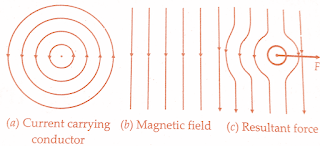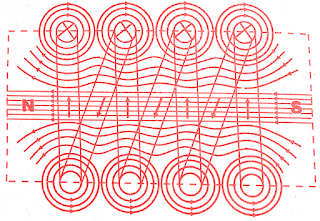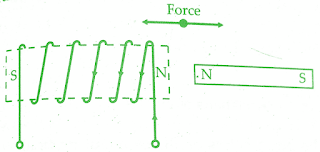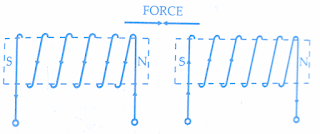Analog Instruments | Classification of Analog Instruments
An analog instrument is one in which the output or display is a continuous function of time and bears a constant relation to its input. Analog instruments find extensive use in present day applications although digital instruments are increasing in number and applications.

The areas of application which are common to both analog and digital instruments are fairly limited at present. Hence, it can safely be predicted that the analog instruments will remain in extensive use for a number of years and are not likely to be completely replaced by digital instruments for certain applications.

Broadly, the analog instruments (and for that matter digital instruments) may be classified according to the quantity they measure.For example, an instrument meant for measurement of current is classified as an Ammeter while an instrument that measures voltage is classified as a Voltmeter. In addition to above instruments, we have wattmeters, power factor meters, frequency meters etc.

Electrical measuring instruments may also be classified according to the kind of current that can be measured by them.Electrical measuring instruments may be classified as instruments for :

(i) direct current (d.c.)
(ii) alternating current (a.c.)
(iii)both direct and alternating current instruments (d.c./a.c.), these instruments are appropriately called universal instruments.

Analog instruments depend for their operation on one of the many effects produced by current and voltage and thus analog instruments are classified according to which of the effects is used for their working.The various effects used are listed in the table present in the middle of this article.

1.Indicating
2.Recording
3.Integrating

Indicating instruments are those instruments which indicate the magnitude of a quantity being measured.They generally make use of a dial and a pointer for this purpose.Ordinary voltmeters, ammeters and wattmeters belong to this category.

(i) electromechanical instruments,
(ii) electronic instruments.

Electronic instruments are constructed by addition of electronic circuits to electromagnetic indicators in order to increase the sensitivity and input impedance.

Recording instruments give a continuous record of the quantity being measured over a specified period. The variations of the quantity being measured are recorded by a pen (attached to the moving system of the instrument ; the moving system is operated by the quantity being measured) on a sheet of paper fixed or moving. For example, we may have a recording voltmeter in a sub-station which keeps a record of the variations of supply voltageduring the day.

Integrating instruments totalize events over a specified period of time. The summation, which they give is the product of time and an electrical quantity. Ampere hour and watt-hour (energy) meters are examples of this category. The integration (summation value) is generally given by a register consisting of a set of pointers and dials. The analog instruments may also be classified on the basis of the method used for comparing the unknown quantity (measured) with the unit of measurement.The two categories of instruments based upon this classification are

#### 1. Direct measuring instruments:

These instruments convert the energy of the measurand directly into energy that actuates the instrument and the value of the unknown quantity is measured or displayed or recorded directly.The examples of this class of instruments are ammeters, voltmeters, wattmeters and energy meters.

#### 2. Comparison instruments:

These instruments measure the unknown quantity by comparison with a standard. (Direct measuring instruments are the most commonly used in engineering practice because they are the most simple and inexpensive. Also, their use makes the measurement possible in the shortest time.) The examples of comparison type instruments are dc and ac bridges. Comparison type instruments are used in cases where a higher accuracy of measurement is required.

Electrical instruments may also be classified according to their accuracy class. The limits of intrinsic error in the measured quantity for instruments for various classes of accuracy are :

### Classification based on principles of operation:

As mentioned earlier secondary analog instruments may be classified according to the principle of operation they utilise. The effects they utilise are :
(i) magnetic effect
(ii) heating effect
(iii) electrostatic effect
(iv) electromagnetic effect
(v) hall effect.

#### 1. Magnetic effect:

Consider a current carrying conductor as shown in below figure(a), it produces a magnetic field in the anticlockwise direction. We now have a uniform magnetic field as shown in below figure(b). Let the current carrying conductor be placed in this magnetic field. The resultant field is as shown in below figure(c). This results in distortion of the magnetic field causing a force F to act from left to right. The reversal of the direction of the current will cause a force F in the opposite direction,i.e., from right to left subject to the condition that the direction of the existing field remains the same.Force produced due to a current carrying a magnetic field

If we form the conductor into a coil, the magnetic field produced by each turn of the coil will add up and the coil will behave as an imaginary magnet as shown in the below figure.Magnetic field produced by current carrying coils
Force of attraction or repulsion: Consider a current carrying coil as shown in the figure above. It produces an imaginary bar magnet. When a piece of soft iron which has not been previously magnetised is brought near the end of the coil, it will be attracted by the coil. Therefore, if we pivot the soft iron on a spindle between two bearings and a coil is mounted near it, the iron piece will swing into the coil when the latter is carrying current. The effect is utilised in the "attraction type of moving iron instruments."

If we have two pieces of soft iron placed near the coil the two will be similarly magnetised and there will be a force of repulsion between them. This effect is utilised in "repulsion type moving iron instruments".

Force between a current carrying coil and a permanent magnet:

Consider the coil as shown in below figure.It produces an imaginary bar magnet when carrying current. When a permanent magnet is brought near it,   there will be either a force of attraction or repulsion. If the coil is mounted on a spindle between bearings, there will be a movement of the coil. This effect is utilised in permanent magnet moving coil instruments

Force between two current carrying coils:

Consider two current carrying coils as shown in the below figure. For the directions of the currents shown, the two produce unlike poles near each other and thus there is a force of attraction and if one of the coils is movable and the other is fixed, there will be a motion of the movable coil. This effect is utilised in the dynamometer type of instruments.Force between current carrying coil and a permanent magnet

#### 2. Thermal effect:

The current to be measured is passed through a small element which heats it. The temperature rise is converted to an emf by a thermocouple attached to the element. A thermocouple consists of lengths of two dissimilar electric conductors joined at ends to form a closed loop. If the junctions of the two dissimilar metals are maintained at different temperatures, a current flows through the closed loop. This current can be measured and is indicative of the r.m.s. value of the current flowing through the heater elements.Force between two current carrying coils

#### 3. Electrostatic effect:

When two plates are charged, there is a force exerted between them. This force is used to move one of the plates. The instruments working on this principle are called electrostatic instruments and they are usually voltmeters.

#### 4. Induction effect:

When a non-magnetic conducting pivoted disc or a drum is placed in a magnetic field produced by a system of electromagnets excited by alternating currents, an emf is induced in the disc or drum. if a closed path is provided, the emf forces a current to flow in the disc or drum.The force produced by the interaction of induced currents and the alternating magnetic fields makes the disc move.The induction effect is mainly utilised in a.c. energy meters.

#### 5. Hall effect:

If a strip of conducting material carries current in the presence of a transverse magnetic field as shown in the below figure, an emf is produced between two edges of the conductor. The magnitude of the voltage depends upon the current, flux density and a property of conductor called "Hall Effect Co-efficient".
The emf may be measured after amplification.Hall effect elements are extensively used in magnetic measurements. They can also be used for sensing of current.Another instrument that uses Hall effect is Poynting Vector Wattmeter.This wattmeter used for measuring the power loss density at the surface of a magnetic material.

The below table gives effects utilised by various types of instruments.

 Effect Instruments Magnetic effect Ammeters,Voltmeters,wattmeters,integrating meters Heating effect Ammeters, Voltmeters, Wattmeters Electrostatic effect Voltmeters Induction effect A.C Ammeters, Voltmeters, Wattmeters, Energy meters Hall effect Flux meters, ammeters and Poynting vector wattmeter

#### Conclusion:

Now here we have discussed the classification of analog measuring instruments.You can download this as pdf,ppt.

Comment below for any Queries.